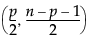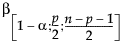Yi is the data for the ith row
Y is the row of means
n = number of observations
p = number of variables (columns)= (1–α)th quantile of a Betadistribution
n = number of observations
p = number of variables (columns)
Mi = Mahalanobis distance for the ith observation
T2 Distance Measures
The T2 distance is the square of the Mahalanobis distance, so Ti2 = Mi2.
The UCL on the T2 distance is:
n = number of observations
p = number of variables (columns)= (1–α)th quantile of a Betadistribution

Help created on 9/19/2017